﻿ An Alternative Derivation of the Fernández-Castro Analytic Approximate Expression for the Eigenvalues of the Bounded Quartic OscillatorInternational Journal of Theoretical and Mathematical Physics

p-ISSN: 2167-6844    e-ISSN: 2167-6852

2018;  8(2): 47-51

doi:10.5923/j.ijtmp.20180802.03### An Alternative Derivation of the Fernández-Castro Analytic Approximate Expression for the Eigenvalues of the Bounded Quartic Oscillator

Department of Physics and Engineering Physics, Obafemi Awolowo University, Ile-Ife, Nigeria

Correspondence to: Kunle Adegoke, Department of Physics and Engineering Physics, Obafemi Awolowo University, Ile-Ife, Nigeria.
 Email: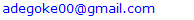Abstract

In this note we show that the standard Rayleigh-Schrödinger (RS) perturbation method gives the same result as the hypervirial pertubative method (HPM), for an approximate analytic expression for the energy eigenvalues of the bounded quartic oscillator. This connection between the HPM and the RS method went unnoticed for a long time, apparently because it was not obvious that the resulting polygamma sums to be evaluated in the RS method could, in fact, be expressed in closed form.

Keywords: Rayleigh-Schrödinger perturbation, Hypervirial pertubative method, Polygamma sum

Cite this paper: Kunle Adegoke, Adenike Olatinwo, Olugbenga Olunloyo, An Alternative Derivation of the Fernández-Castro Analytic Approximate Expression for the Eigenvalues of the Bounded Quartic Oscillator, International Journal of Theoretical and Mathematical Physics, Vol. 8 No. 2, 2018, pp. 47-51. doi: 10.5923/j.ijtmp.20180802.03.

### 1. Introduction

Interest in the bounded quartic oscillator started with the pioneering work of Barakat and Rosner  who employed a power series method to obtain numerical values of the eigenvalues through an iteration scheme. Researchers have since continued to investigate the bounded quartic oscillator and related systems, using various techniques (see references [2, 3, 4, 5] and the references in them).
The bounded quartic oscillator is described by the Hamiltonian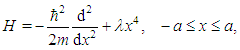(1)
where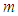is the mass of the oscillator and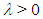is the coupling constant. The Hamiltonian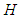lives in a Hilbert space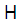with inner product between any two real-valued functions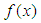and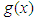in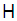defined by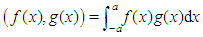, where the functions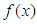and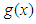and indeed all vectors of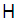are required to vanish at the boundaries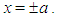About three and a half decades ago, using their hypervirial pertubative method (HPM), Fernández and Castro  derived the following expression (their equation (22) in our notation) for the eigenvalues of the bounded quartic oscillator: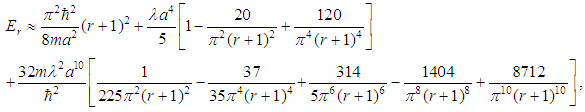(2)
for quantum numbers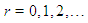In this paper we show that the standard Rayleigh-Schrödinger (RS) perturbation theory with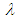as the perturbation parameter gives the same result for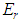as given in (2). As a matter of fact we stumbled upon the work of Fernández and Castro only after we had obtained our result for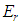. The Computer Algebra System Waterloo Maple came to our aid in simplifying the resulting perturbation sums and finding their closed form.

### 2. Basis Functions and the Matrix Elements of H

Since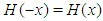, the eigenstates of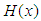have definite parity. For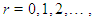the complete orthonormal functions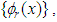where,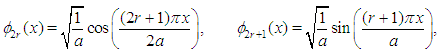constitute a suitable set of basis functions in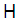for a matrix representation of the bounded quartic oscillator Hamiltonian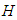, since they also satisfy the boundary conditions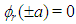.
The identities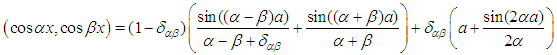and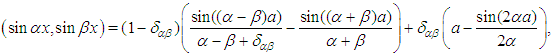for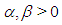and the repeated application of Leibnitz rule for differentiating an integral allow to calculate the matrix elements of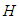as(3)
provided that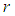and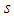have the same parity, and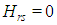otherwise.
The matrix elements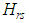facilitate the direct diagonalization of the bounded quartic oscillator. The energy eigenvalues can be made arbitrarily accurate by increasing the dimension of the Hamiltonian matrix used; the eigenvalues obtained can therefore be considered exact. We are, however, not concerned here with exact diagonalization but we need the matrix elements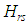for our perturbation calculations.
For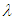sufficiently small (see  for a rigorous discussion of the convergence criteria), the oscillator potential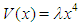may be treated as a perturbation of the unperturbed Hamiltonian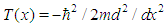(the free particle in a box Hamiltonian).
In the standard Rayleigh-Schrödinger perturbation theory for non-degenerate states, the approximate energy eigenvalues of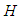, to second order in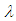, are to be calculated from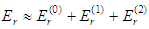. We have immediately that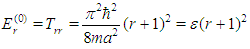(4)
and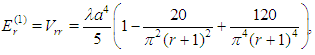(5)
where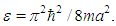The second order correction to the energy of the bounded quartic oscillator,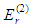, is given by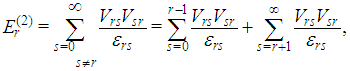(6)
where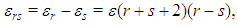(7)
so that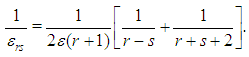(8)
Since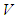is a real symmetric matrix, (6) is simply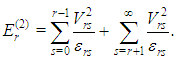(9)
We note that the matrix elements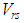occuring in (9) are necessarily off-diagonal (since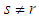). Furthermore the only surviving elements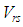, according to (3), are those for which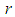and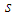are both odd or both even.
It therefore follows from (3) that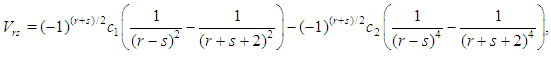(10)
where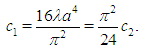Taking (8) into account, the summand in (9) is therefore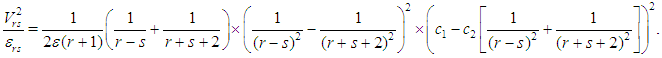(11)
The sum in (9) is easier to evaluate if the energy eigenvalues are grouped by parity: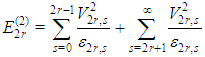and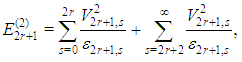for quantum number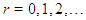Using the summation identity (equation 2.6 of )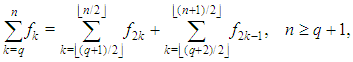wheredenotes the floor of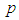, that is, the greatest integer less than or equal to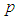, the above sums can be expressed as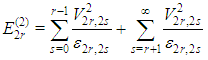(12)
and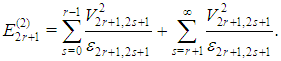(13)
Maple is able to evaluate the sums in (12) and (13), with the appropriate summand in each case obtained from (11), and we have (see the Maple code in the appendix)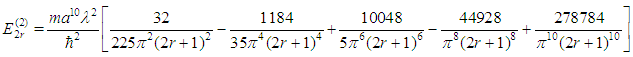and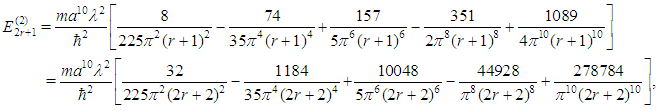from which it follows that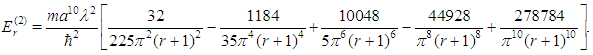(14)
Adding (4), (5) and (14), we finally obtain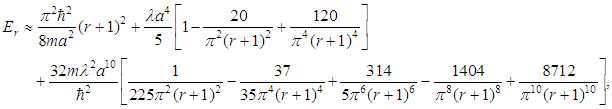as an approximate expression for the eigenvalues of the bounded quartic oscillator.

### 3. Summary and Conclusions

Using the Rayleigh-Schrödinger perturbation theory and with the aid of a summation identity and the Computer Algebra System Maple, we have derived an approximate expression for the energy eigenvalues of the bounded quartic oscillator. This is the same expression that was obtained much earlier in reference  through a more complicated approach. Similar results to ours are also contained in reference  where exact diagonalization was done and perturbative series up to the third order in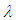were also developed for the energy levels. However, the RS sums were determined numerically in that paper as, apparently, closed form could not be found for them; and furthermore only results for the ten lowest eigenvalues were computed.

### Appendix

Maple code to evaluate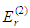>V:=(r,s)->(-1)^(r+s)*c1*(1/(r-s)^2-1/(r+s+2)^2) -(-1)^(r+s)*c2*(1/(r-s)^4-1/(r+s+2)^4):
>epsilon:=(r,s)->epsilon*(r+s+2)*(r-s):
>simplify(expand(eval(V(r,s)^2/epsilon(r,s),[r=2*r,s=2*s]))) assuming r,posint,s,posint,c1>0,c2>0:
> summand:=convert(
>S1:=sum(summand,s=0..r-1) assuming r,posint,s,posint,c1>0,c2>0:
>S2:=sum(summand,s=r+1..infinity) assuming r,posint,s,posint,c1>0,c2>0:
> ssum:=simplify(S1+S2):
>fsum:=expand(eval(ssum,[epsilon=Pi^2*h^2/8/m/a^2, c1=16*lambda*a^4/Pi^2,c2=16*24*lambda*a^4/Pi^4])):
>arranged:=collect(fsum,Pi):
>terrms:=[seq(factor(op(i,arranged)),i=1..nops(arranged))]: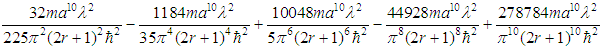R. BARAKAT AND R. ROSNER (1981), The bounded quartic oscillator, Physics Letters 83A (4):149--150.  F. M. FERNÁNDEZ and E. A. CASTRO (1982), An analytic approximate expression for the eigenvalues of the bounded quartic oscillator, Physics Letters 88A (1):4--6.  V. C. AGUILERA-NAVARRO, J. F. GOMES and A. H. ZIMERMAN (1983), On the quantum quartic oscillator in a box, Revista Brasileira de Fisica 13 (4):664--672.  R. N. CHAUDHURI AND B. MUKHERJEE (1983), The eigenvalues of the bounded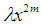oscillators, Journal of Physics A: Math. Gen. 16:3193--3196.  H. A. ALHENDI AND E. I. LASHIN (2005), Spectrum of one-dimensional anharmonic oscillators, Canadian Journal of Physics 83:541--550.  H. W. GOULD (2011), Table for Fundamentals of Series: Part I: Basic properties of series and products, From the seven unpublished manuscripts of H. W. Gould.